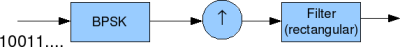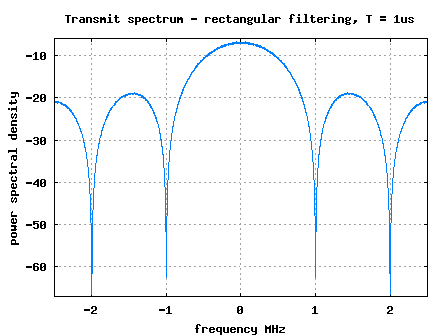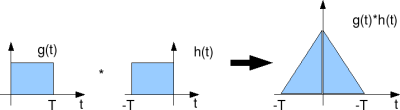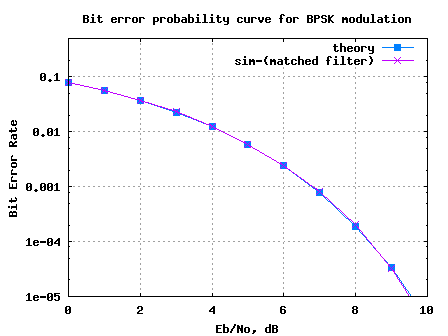- DSP log - http://www.dsplog.com -

BER with Matched Filtering

Posted By Krishna Sankar On May 8, 2009 @ 5:40 am In Channel | 38 Comments

In the post on transmit pulse shaping filter , we had discussed pulse shaping using rectangular and sinc. In this post we will discuss about optimal receiver structure when pulse shaping is used at the transmitter. The receiver structure is also called as matched filter. For the discussion, we will assume rectangular pulse shaping, the channel is AWGN only and the modulation is BPSK.

## Transmitter

The simplified transmitter structure is as shown below:Transmit pulse shaping filter

Figure: Transmit block diagram

The sequence of operation are as follows:

a). The random binary data comes as input to the the BPSK modulator circuit.

b). The BPSK modulator maps bits to symbols i.e bit0 to -1 and bit1 to +1

The sequence of transmit symbols maybe visualized as follows.

Figure: Transmit symbols for baseband PAM transmission

c). The upsampling block (the block with upward arrow) insert zeros between the samples based on the oversampling factor. For example, if the oversampling factor is 4, the block inserts 3 zeros between samples.

d). The last block in the chain is the filter, where the upsampled sequence is convolved with the filter. In this example, we have assumued rectangular filtering (also known as zero order hold), i.e. to repeat the current symbol till the next symbol arrives and so on. Mathematically, the filter can be represented as,

.

The filtered waveform can be as shown below.

Figure: Baseband PAM transmisison with rectangular filtering

The transmit spectrum assume that the symbol duration is shown below.Figure: Transmit spectrum for rectangular matched filter

The filter having an impulse response is called a matched filter of .Matched filter response for rectangular pulse

Figure: Matched filter response for a rectangular pulse shaping filter

In this example, as is a rectangular function, the mtched filter is also rectangular.

We pass the received signal through the matched filter . The output of the convolution peaks at time and we sample the at that time instant to perform the demodulation.

Further, if the filtered transmit signal is corrupted by noise, the filter with impulse response matched to maximizes the output Signal to Noise Ratio. The proof for this claim is detailed in Chapter 5.1.2 Matched Filter Demodulator in Digital Communications by John Proakis. 

## Simulation Model

The Matlab/Octave script performs the following

(a) Generate random binary sequence of +1′s and -1′s.

(b) Upsample by inserting zeros and the convolve with rectangular filter

(d) Convolve the received samples with matched filter, and extract output at time T

(e) Perform hard decision decoding and count the bit errors

(f) Repeat for multiple values of and plot the simulation and theoretical results.Figure: BER plot for BPSK with rectangular pulse shaping and matched filtering

## Observations

Can see that the simulated results are in good agreement with the theoretical BER results for BPSK in AWGN in AWGN .

## References

Digital Communications by John Proakis.URL to article: http://www.dsplog.com/2009/05/08/ber-with-matched-filtering/

URLs in this post:

 transmit pulse shaping filter: http://www.dsplog.com/2008/04/14/transmit-pulse-shape-nyquist-sinc-rectangular/